# “MobileNetV2: Inverted Residuals and Linear Bottleneck” Summarized

Categories:

Updated:

https://arxiv.org/abs/1801.04381 (2019-3-21)

## 1. Introduction

This paper introduces a new neural network architecture that is specifically tailored for mobile and resource constrained environments. Its main contribution is a novel layer module: the inverted residual with linear bottleneck.

MobileNetV2 can be efficiently implemented using standard operations in any modern framework and beats state of the art along multiple performance points using standard benchmarks. Also, it significantly reduces the memory footprint needed during inference by never fully materializing large intermediate tensors.

## 2. Depthwise Separable Convolutions

The basic idea is to replace a full convolutional operator with a factorized version that splits convolution into two separate layers.

1. depthwise convolution: it performs lightweight filtering by applying a single convolutional filter per input channel.
2. pointwise convolution: 1x1 convolution that builds new features through computing linear combinations of the input channels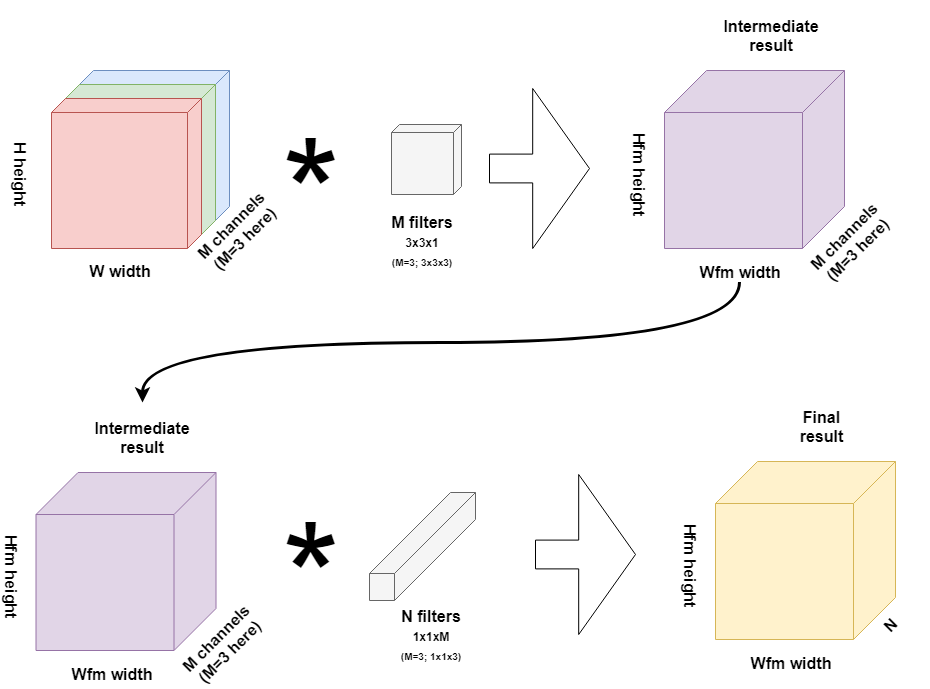Standard convolutional layers have the computational cost of $h_iw_id_id_j k^2$ where $h_i, w_i, d_i$ are the height, width, depth of the input tensor, $d_j$ is the depth of the output tensor, and $k$ is the size(width=height) of the convolutional kernel. On the other hand, depthwise separable convolutions empirically work almost as well as regular convolutions but only cost $h_iw_id_i(k^2+d_j)$.

So depthwise convolution reduces computation by almost a factor of $k^2$. MobileNetV2 uses $k=3$ so the computational cost is 8 to 9 times smaller than that of standard convolutions at only a small reduction in accuracy.

## 3. Linear Bottlenecks

We say that the set of layer activations forms a ‘manifold of interest’. It has been long assumed that manifold of interest in neural networks could be embedded in low-dimensional subspace.

Then why shouldn’t we just reduce the dimensionality of a layer? Actually this has been successfully exploited by MobileNetV1. It uses width multiplier(that is multiplied to baseline depth in order to scale the network) to reduce the dimensionality of the activation space until the manifold of interest spans this entire space.

However, this intuition breaks when we recall that deep convolutional neural networks actually have non-linear per coordinate transformation, a ReLU. In other words, deep networks only have the power of a linear classifier on the non-zero volume part of the output domain.

On the other hand, when ReLU collapses the channel, it inevitably loses information in that channel. However if we have lots of channels and there is a structure in the activation manifold, that information might still be preserved in the other channels.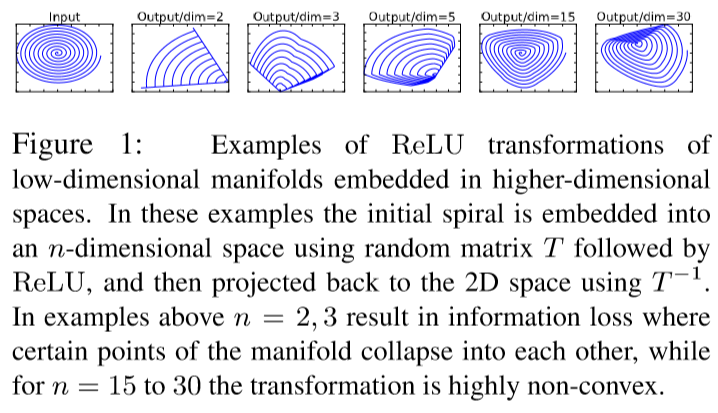To summarize, there are two properties that are indicative of the requirement that the manifold of interest should lie in a low-dimensional subspace of the higher-dimensional activation space:

1. If the manifold of interest remains non-zero volume after ReLU transformation, it corresponds to a linear transformation.
2. ReLU is capable of preserving complete information about the input manifold, but only if the input manifold lies in a low-dimensional subspace of the input space.

In conclusion, if we assume the manifold of interest is low-dimensional we can capture this by inserting linear bottleneck layers into the convolutional blocks. Experimental evidence suggests that using linear layers is crucial as it prevents nonlinearities from destroying too much information.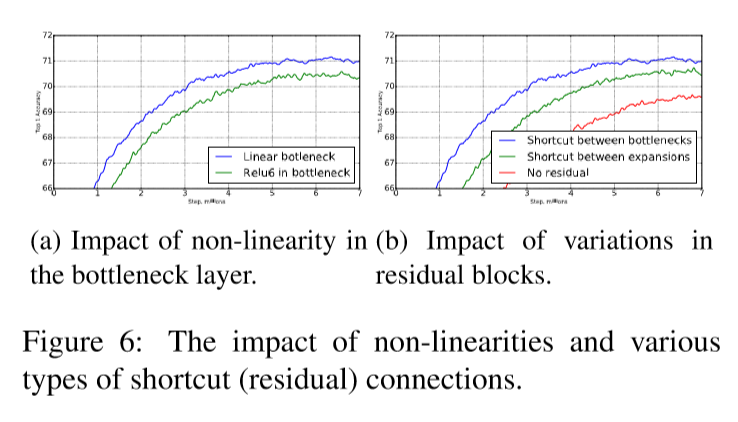## 4. Inverted Residuals

Original residual block contains an input followed by several bottlenecks then followed by expansion, and the shortcuts exist between thick(has many channels) layers. However, inspired by the intuition that the bottlenecks actually contain all the necessary information and expansion layer acts merely as a non-linear transformation, MobileNetV2 uses shortcuts directly between the bottlenecks(thin layers).

This ‘inverted’ design is considerably more memory efficient, and also works sightly better than conventional residual blocks.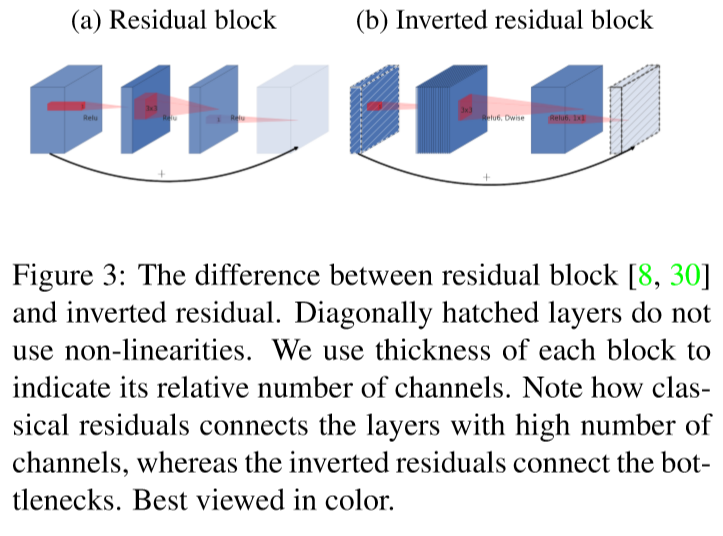Below image illustrates how the concepts of 1) separable convolution, 2) linear bottleneck, and 3) inverted residual evolved.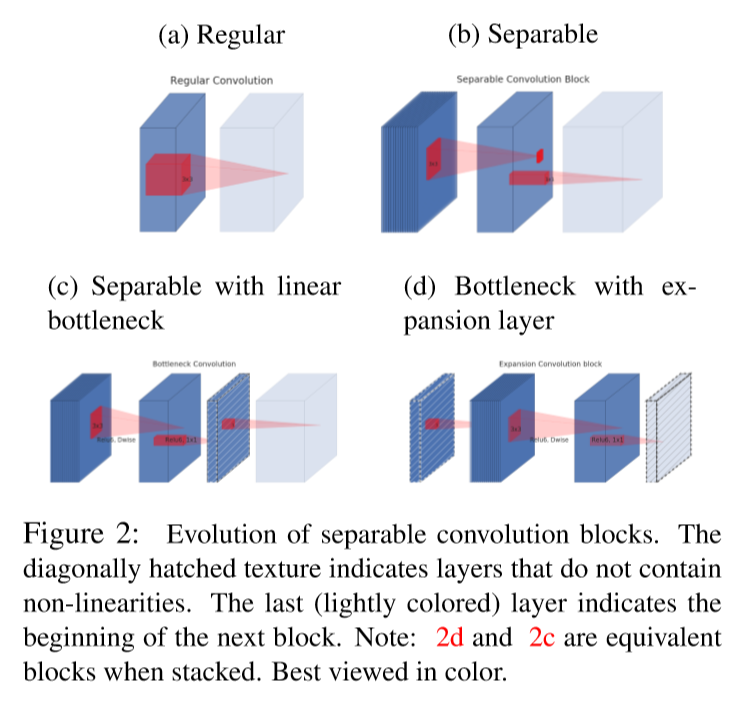## 5. Information Flow Interpretation

MobileNetV2 provides a natural separation between two things, which have been tangled together in previous nn architectures.

1. Capacity: input/output domains of the building blocks / encoded by bottleneck inputs
2. Expressiveness: layer transformation (non-linear function that converts input to the output) / encoded by expansion layers

Authors say that exploring these concepts separately is an important direction for future research.

## 6. Architecture Details

• Used ReLU6 (min(max(x, 0), 6)) as the non-linearity because of its robustness when used with low-precision computation
• Used 6 as the expansion rate(multiplied to the input channels to form the middle non-linear convolutional layers).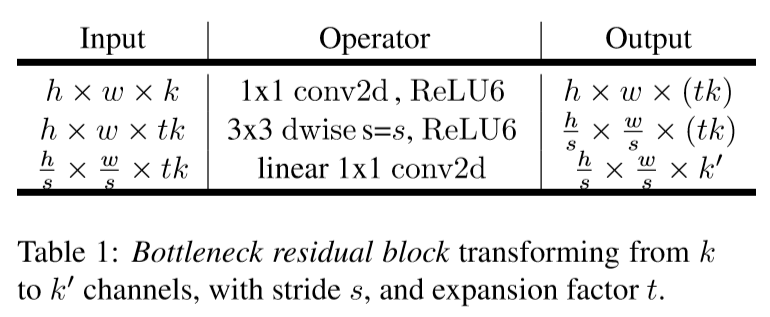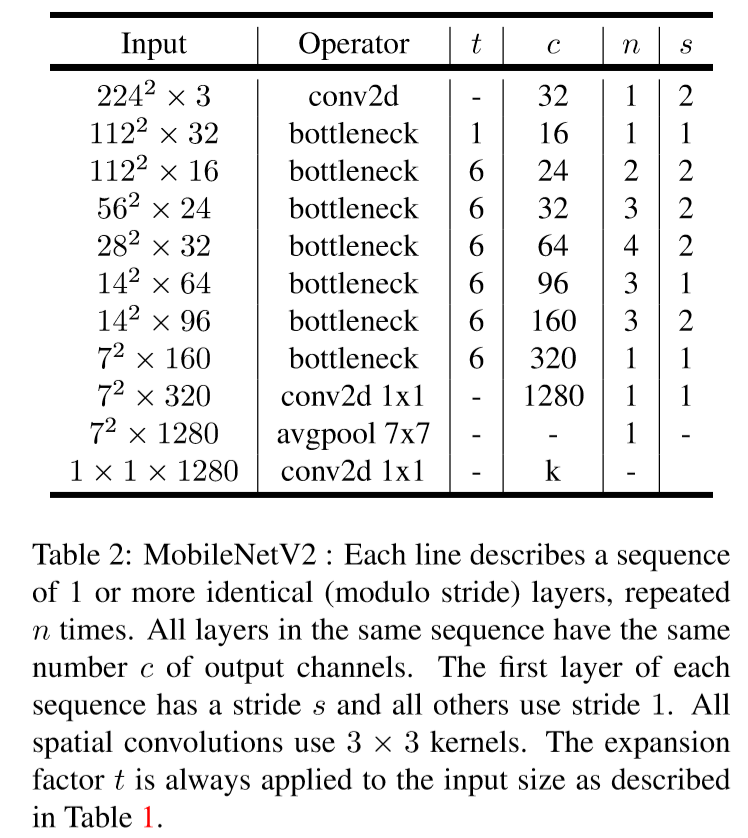## 7. Memory Efficient Inference

A bottleneck block operator $F(x)$ can be expressed as a composition of three operators:

where $N=ReLU6 \circ dwise \circ ReLU6$.

Inner tensor can be represented as concatenation of $t$ tensors, of size $n/t$ each:

Consequently, we only require one intermediate block of size $n/t$ to be kept in memory at all times.

The two constraints that enabled us to use this trick is:

1. The inner transformation is per-channel.
2. The consecutive non-per-channel operators have significant ratio of the input size to the output.

However, replacing one matrix multiplication with several smaller ones hurts runtime performance due to increased misses. The authors find that setting $t$ between 2 and 5 worked best.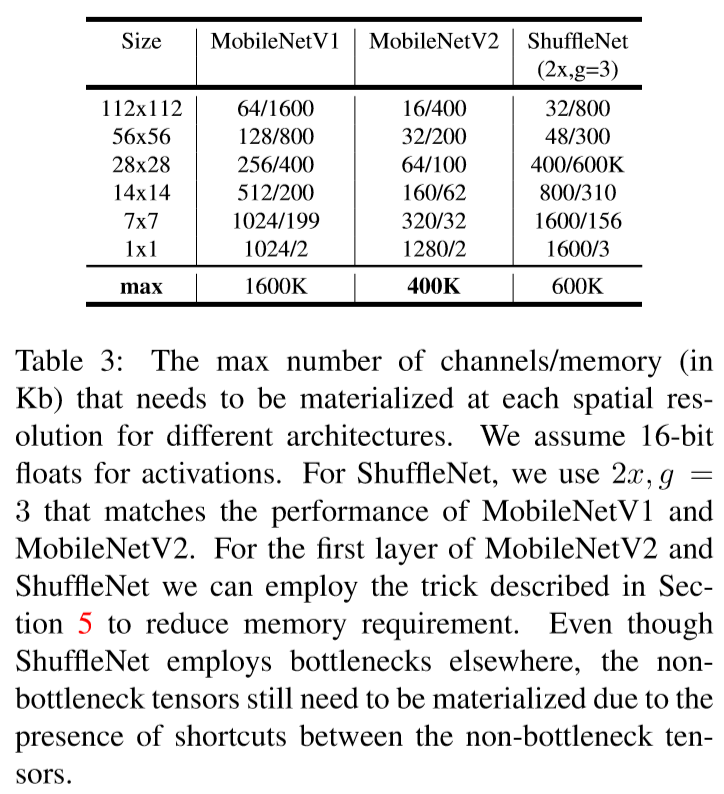## 8. Experiment Results

### 1) ImageNet Classification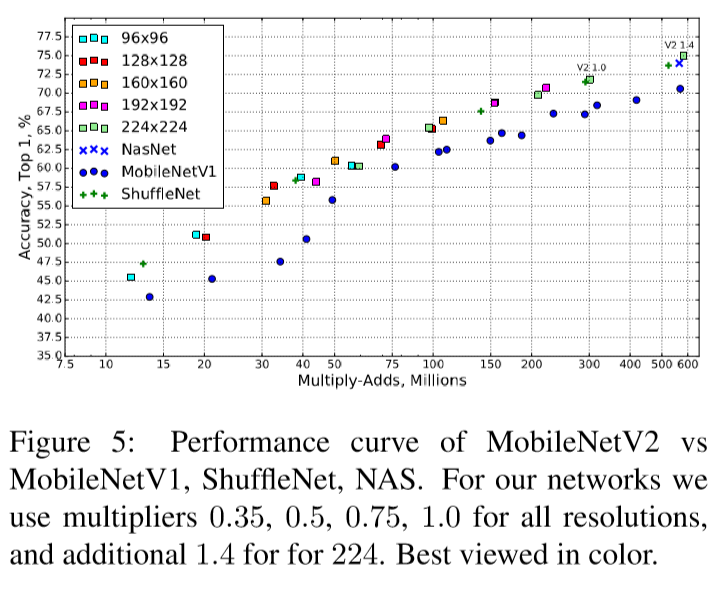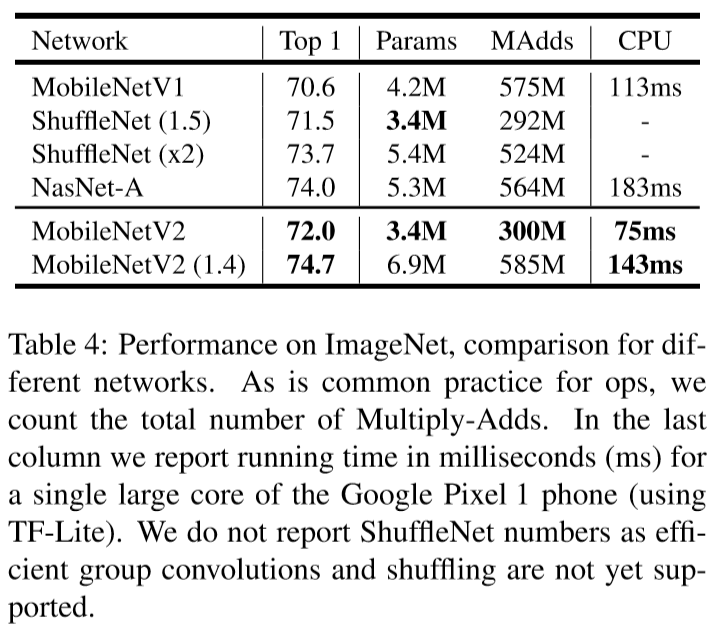### 2) Object Detection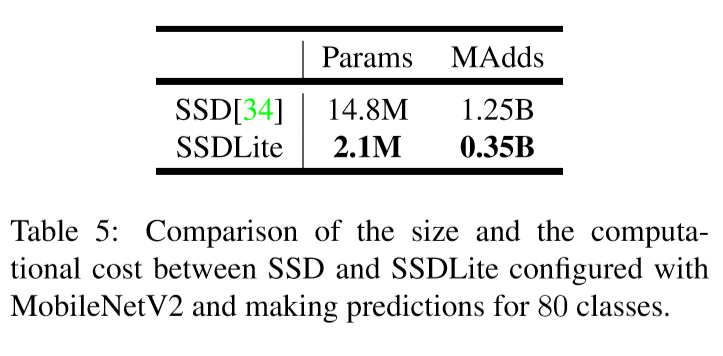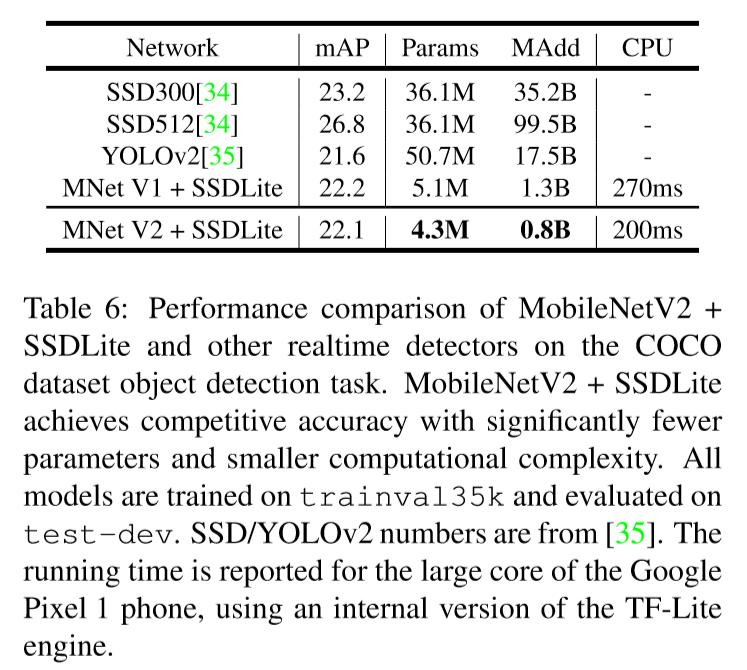### 3) Semantic Segmentation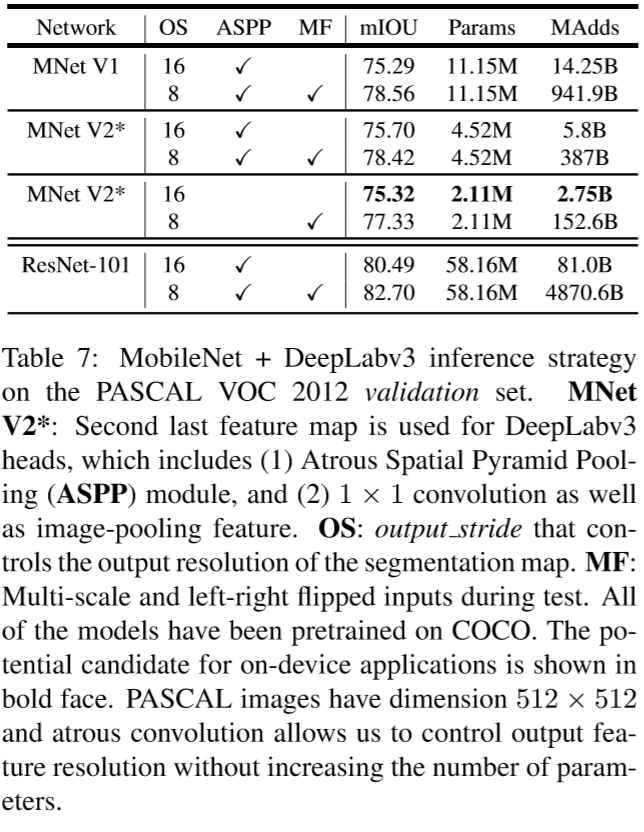Categories: